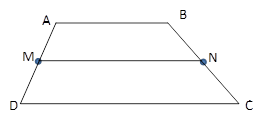Chapter 4.4, Problem 12EElementary Geometry For College St...

7th Edition
Alexander + 2 others
ISBN: 9781337614085

Solutions

Chapter
SectionElementary Geometry For College St...

7th Edition
Alexander + 2 others
ISBN: 9781337614085
Textbook Problem

In Exercises 11 to 16, the drawing shows trapezoid ABCD with A B ¯ | | D C ¯ ; also, M and N are midpoints of A D ¯ and B C ¯ , respectively.Exercises 11-16Given: M N = 6.3   a n d   D C = 7.5 Find: A B

To determine

To Find:

AB of trapezoid ABCD.

Explanation

Consider the following trapezoid ABCD.

Given:

MN=6.3 and DC=7.5

In the given trapezoid, AB¯||DC¯.

M is the midpoint of the side AD¯ and N is the midpoint of the side BC¯

Still sussing out bartleby?

Check out a sample textbook solution.

See a sample solution

The Solution to Your Study Problems

Bartleby provides explanations to thousands of textbook problems written by our experts, many with advanced degrees!

Get Started

A sample of n = 9 scores has X = 108. What is the sample mean?

Essentials of Statistics for The Behavioral Sciences (MindTap Course List)

Convert the expressions in Exercises 6584 to power form. x23

Finite Mathematics and Applied Calculus (MindTap Course List)

True or False: The slope of a tangent line may be interpreted as average velocity.

Study Guide for Stewart's Single Variable Calculus: Early Transcendentals, 8th